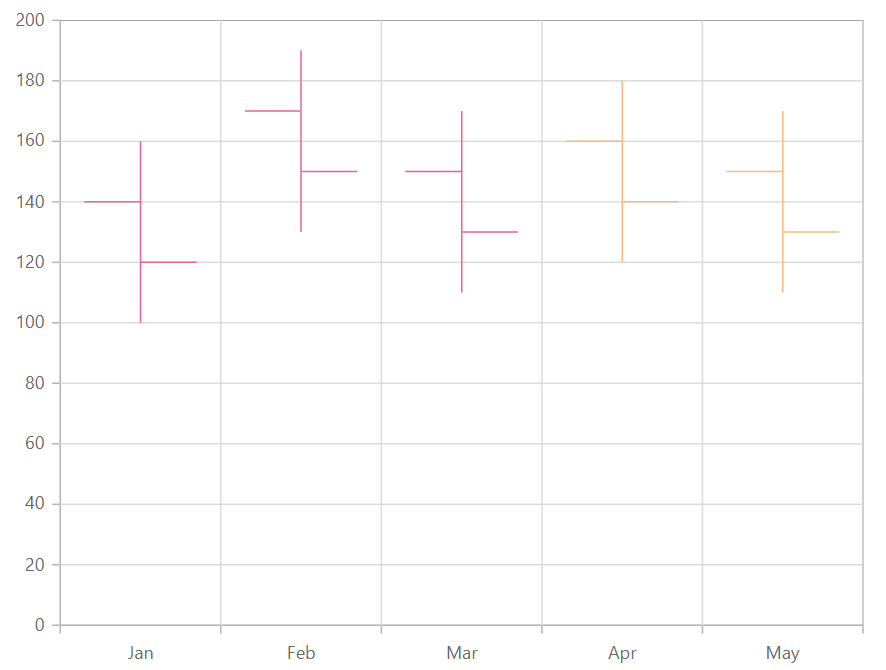# High Low Open Close in Blazor Charts Component

14 Aug 20233 minutes to read

## High Low Open Close

HiloOpenClose series is used to represent the Low, High, Open and Closing values over time and it can be rendered by specifying the series Type as HiloOpenClose.

``````@using Syncfusion.Blazor.Charts

<SfChart>
<ChartPrimaryXAxis ValueType="Syncfusion.Blazor.Charts.ValueType.Category" />

<ChartSeriesCollection>
<ChartSeries DataSource="@StockDetails" XName="X" High="High" Low="Low" Open="Open" Close="Close" Type="ChartSeriesType.HiloOpenClose">
</ChartSeries>
</ChartSeriesCollection>
</SfChart>

@code{
public class Data
{
public string X { get; set; }
public double Y { get; set; }
public double High { get; set; }
public double Low { get; set; }
public double Open { get; set; }
public double Close { get; set; }
}

public List<Data> StockDetails = new List<Data>
{
new Data{ X= "Jan", Open= 120, High= 160, Low= 100, Close= 140 },
new Data{ X= "Feb", Open= 150, High= 190, Low= 130, Close= 170 },
new Data{ X= "Mar", Open= 130, High= 170, Low= 110, Close= 150 },
new Data{ X= "Apr", Open= 160, High= 180, Low= 120, Close= 140 },
new Data{ X= "May", Open= 150, High= 170, Low= 110, Close= 130 }
};
}``````

## Series customization

In HiloOpenClose series, BullFillColor property is used to fill the segment when the open value is greater than the close value and BearFillColor property is used to fill the segment when the open value is less than the close value. By default, BullFillColor is set as green and BearFillColor is set as red.

``````@using Syncfusion.Blazor.Charts

<SfChart>
<ChartPrimaryXAxis ValueType="Syncfusion.Blazor.Charts.ValueType.Category"/>

<ChartSeriesCollection>
<ChartSeries DataSource="@StockDetails" XName="X" High="High" Width="5" BearFillColor="darkred" BullFillColor="darkgreen" Low="Low" Open="Open" Close="Close" Type="ChartSeriesType.HiloOpenClose">
</ChartSeries>
</ChartSeriesCollection>
</SfChart>

@code{
public class Data
{
public string X { get; set; }
public double Y { get; set; }
public double High { get; set; }
public double Low { get; set; }
public double Open { get; set; }
public double Close { get; set; }
}

public List<Data> StockDetails = new List<Data>
{
new Data{ X= "Jan", Open= 120, High= 160, Low= 100, Close= 140 },
new Data{ X= "Feb", Open= 150, High= 190, Low= 130, Close= 170 },
new Data{ X= "Mar", Open= 130, High= 170, Low= 110, Close= 150 },
new Data{ X= "Apr", Open= 160, High= 180, Low= 120, Close= 140 },
new Data{ X= "May", Open= 150, High= 170, Low= 110, Close= 130 }
};
}``````NOTE

Refer to our Blazor Charts feature tour page for its groundbreaking feature representations and also explore our Blazor Chart Example to know various chart types and how to represent time-dependent data, showing trends at equal intervals.